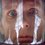# Playing with Integrals: Limits and Integrals 2

I would like to continue the discussion started in this post by offering you a set of even more challenging limits. (As an additional exercise try solving these limits without Riemann Sums) Lets proceed to our first not so trivial example.

Problem 4. Find the following limit $\lim_{n\to\infty}\frac{1}{n}\sqrt[n]{(n+1)(n+2)...(2n)}$

Solution. We can't apply our tactic directly because we have to deal with product rather than sum. However, as many of you can guess, there is a natural way to transform the product into sum. $\lim_{n\to\infty}\frac{1}{n}\sqrt[n]{(n+1)(n+2)...(2n)}=\lim_{n\to\infty}\sqrt[n]{\prod^n_{i=1}\left(1+\frac{i}{n}\right)}=$ $=\lim_{n\to\infty}{\prod^n_{i=1}\left(1+\frac{i}{n}\right)}^{\frac{1}{n}}=\lim_{n\to\infty}e^{\frac{1}{n}\sum^n_{i=1}\ln\left(1+\frac{i}{n}\right)}$

Now we see a familiar construction, which can be easily evaluated, namely $\lim_{n\to\infty}\frac{1}{n}\sum^n_{i=1}\ln\left(1+\frac{i}{n}\right)=\int^1_0\ln(1+x)\,dx=\ln4 - 1.$

Now returning to the initial problem we get $\lim_{n\to\infty}\frac{1}{n}\sqrt[n]{(n+1)(n+2)...(2n)}=\lim_{n\to\infty}e^{\frac{1}{n}\sum^n_{i=1}\ln\left(1+\frac{i}{n}\right)}=$ $=e^{\ln4 - 1}=\boxed{\frac{4}{e}}.$

Almost the same problem was proposed at National Mathematics Olympiad in Moldova in 2007.

Problem 5. (Moldova, 2007, $12^{th}$ class) Calculate the limit $\lim_{n\to\infty}\frac{\sqrt[n+1]{(2n+3)(2n+4)...(3n+3)}}{n+1}$

I also would like to propose another problem from Moldova, this time it's District Mathematics Olympiad, 2013. It's much easier than examples discussed in this post, but still serves a decent technique trainer.

Problem 6. (Moldova, 2013, $12^{th}$ class) Find the limit $\lim_{n\to\infty}\sum^n_{i=1}\frac{n}{(n+1)\sqrt{n(n+i)}}$Note by Nicolae Sapoval
7 years, 5 months ago

This discussion board is a place to discuss our Daily Challenges and the math and science related to those challenges. Explanations are more than just a solution — they should explain the steps and thinking strategies that you used to obtain the solution. Comments should further the discussion of math and science.

When posting on Brilliant:

• Use the emojis to react to an explanation, whether you're congratulating a job well done , or just really confused .
• Ask specific questions about the challenge or the steps in somebody's explanation. Well-posed questions can add a lot to the discussion, but posting "I don't understand!" doesn't help anyone.
• Try to contribute something new to the discussion, whether it is an extension, generalization or other idea related to the challenge.
• Stay on topic — we're all here to learn more about math and science, not to hear about your favorite get-rich-quick scheme or current world events.

MarkdownAppears as
*italics* or _italics_ italics
**bold** or __bold__ bold
- bulleted- list
• bulleted
• list
1. numbered2. list
1. numbered
2. list
Note: you must add a full line of space before and after lists for them to show up correctly
paragraph 1paragraph 2

paragraph 1

paragraph 2

[example link](https://brilliant.org)example link
> This is a quote
This is a quote
    # I indented these lines
# 4 spaces, and now they show
# up as a code block.

print "hello world"
# I indented these lines
# 4 spaces, and now they show
# up as a code block.

print "hello world"
MathAppears as
Remember to wrap math in $$ ... $$ or $ ... $ to ensure proper formatting.
2 \times 3 $2 \times 3$
2^{34} $2^{34}$
a_{i-1} $a_{i-1}$
\frac{2}{3} $\frac{2}{3}$
\sqrt{2} $\sqrt{2}$
\sum_{i=1}^3 $\sum_{i=1}^3$
\sin \theta $\sin \theta$
\boxed{123} $\boxed{123}$

## Comments

Sort by:

Top Newest

Nice post Nicolae! :)

Problem 5:

It can be written as:

$\displaystyle \lim_{n\rightarrow \infty} \exp\left(\frac{1}{n+1}\sum_{i=1}^{n+1}\ln\left(2+\frac{i}{n+1}\right)\right)$

The above is equivalent to:

$\displaystyle \exp\left(\int_0^1 \ln(2+x)\,\,dx\right) = \exp\left(\ln\left(\frac{27}{4}\right)-1\right)=\frac{27}{4e}$

Problem 6:

The given expression is equivalent to:

$\displaystyle \lim_{n\rightarrow \infty} \sum_{i=1}^n \cfrac{1}{n\left(1+\frac{1}{n}\right)\sqrt{1+\frac{i}{n}}}$

As $n\rightarrow \infty$, $1+1/n=1$ and the rest of the expression is same as:

$\displaystyle \int_0^1 \frac{1}{\sqrt{1+x}}dx=2(\sqrt{2}-1)$

- 7 years, 5 months ago

Log in to reply

Good job! Stay tuned for the updates. I plan to write one more post like this and then to end the series on Limits and Integrals with a small test

- 7 years, 5 months ago

Log in to reply

Thank you. :)

I eagerly wait for your upcoming posts.

- 7 years, 5 months ago

Log in to reply

×

Problem Loading...

Note Loading...

Set Loading...